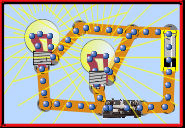# Combined Series and Parallel Circuits: Interactive Lecture Demonstrations

This material is replicated on a number of sites as part of the SERC Pedagogic Service Project

## SummaryThis sequence of interactive lecture-demonstration questions provides a probe of student understanding of fundamental concepts in DC circuits. There are two initial questions, the first about a two-resistor series circuit and the second about a two-resistor parallel circuit. The final question is on a combined circuit with one light bulb in series with two in parallel. Students who understand this circuit should be able to generalize this understanding analyze a large number of different circuit types.

The demonstrations used to motivate these questions can be done either with simple circuit elements, a virtual circuit simulator, or both. Using the virtual simulation will allow the students to perform the experiments themselves outside of class and encourage hands-on exploration of the properties of circuits.

## Learning Goals

The goal of these questions is to judge student understanding of currents and voltages in series, parallel, and combined DC circuits. The questions cover fundamental properties of current, voltage, and the properties of batteries, wires, and resistors. Students must apply their understanding that the current through series circuit elements is equal and the potential difference across parallel circuit elements is equal to solve real-world, non-numerical circuit problems. Seeing results of experiments or simulations motivate conceptual learning. Students must then solve a richer, related, but not obviously identical problem.

## Context for Use

These questions have been used for in-class peer instruction in a calculus based introductory physics course for engineers.

For each question, students should make predictions, share predictions, and discuss and make new predictions. The exercise works best if the students commit to their predictions through either in-class polling or worksheets. There should also be a general class discussion/presentation after each question to clarify the material.

Students should have had an introduction to current, voltage, resistance, and power, either from reading, previous class work, or discussions. Previous reading in series and parallel circuits should be assigned. The author uses this activity as a motivating demonstration at the beginning of the teaching of multi-resistance DC circuits. Other instructors use this as an activity later in the topic to assess understanding.

## Description and Teaching Materials

The demonstration aspects of these questions can be done with either a virtual or a real experimental set up.

The virtual experiment can be done with the PhET Circuit Simulator from the University of Colorado. This is avialable at https://phet.colorado.edu/sims/html/circuit-construction-kit-dc-virtual-lab/latest/circuit-construction-kit-dc-virtual-lab_en.html. The virtual lab has the advantage that students can perform similar experiments if or when they can.

Real experiments can be done using a 6 V dry cell battery, several resistors ranging between 10 ohms and 1,000 ohms, a variable resistor (optional), one or two multimeters that can can be projected for reading by the class, three flashlight bulbs in sockets, and wires as needed to create the circuits.

The questions, descriptions of the misconceptions often found with these questions, and a short description of how to run the labs, are:

## Teaching Notes and Tips

See discussions included with each question.

Students must become engaged in the class activity in order for us to help them learn. Students should commit to an answer before the demonstration, either by written responses or some manner of class-room voting. Peer Instruction, where students can learn from each other before re-voting, has proven to be an effective tool for engaging students.

## Assessment

Qualitative problems similar to the first and third questions can be given on homework and tests. These "What happens when the resistance changes..." questions can probe for understanding. Quantitative problem solving questions where understanding general trends in voltage and current as resistance changes can also be created.

## References and Resources

Results of studies on the effectiveness of PhET simulations, and presentations given about the PhET materials are available at https://phet.colorado.edu/en/research.